## 0x08 随机策略梯度

### 优缺点及分类

• 更好的收敛性
• 更简单的搜索方法
• 可以学到随机策略
• 可能收敛到局部最小，学习不够高效！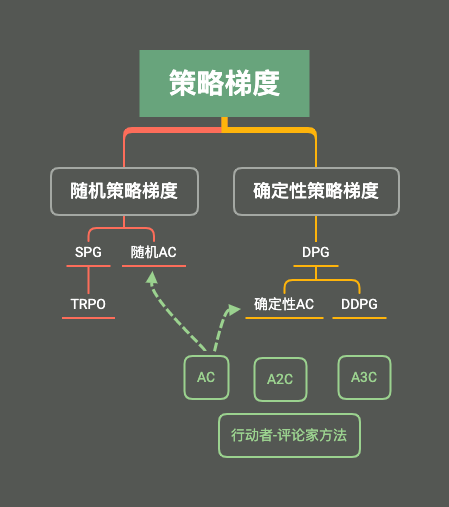### 随机策略梯度定理及证明

• 对于有终止状态的情况，可以使用累计回报作为目标函数，即：

$J(\theta) = V_{\pi_{\theta}}(s_0)$

• 对于没有终止状态的，考虑一段时间的回报期望作为目标函数，即：

$J(\theta) = \sum_{t=1}u_{\pi_{\theta}}(s)\sum_{a}\pi_{\theta}(a|s,\theta)R_s^a$

其中$u_{\pi_{\theta}}(s)$ 是基于策略$\pi_{\theta}$生成的马尔可夫链关于状态的分布，在分布未知的情况下，就算目标函数对策略参数的梯度十分困难！

策略梯度定理能很好解决这种问题，并将上述两种情况在理论上统一成一种表示式：

\begin{aligned} \nabla J(\theta) &\propto \sum_su_{\pi_{\theta}}(s)\sum_aQ_{\pi}(s,a)\nabla_{\theta}\pi_{\theta}(a|s,\theta)\\ &=\sum_s u_{\pi_{\theta}}(s) \sum_a\pi_{\theta}(a|s,\theta)Q_{\pi}(s,a)\frac{\nabla_{\theta}\pi_{\theta}(a|s,\theta)}{\pi_{\theta}(a|s,\theta)}\\ &=E_{s\sim u,a\sim \pi}[Q_{\pi}(s,a)\frac{\nabla_{\theta}\pi_{\theta}(a|s,\theta)}{\pi_{\theta}(a|s,\theta)}]\\ &=E_{s\sim u,a\sim \pi}[\nabla_{\theta}log\pi_{\theta}(a|s,\theta)Q_{\pi}(s,a)] \end{aligned}

### 蒙特卡罗策略梯度

$\nabla_{\theta}J(\theta) =E_{s\sim u,a\sim \pi}[\nabla_{\theta}log\pi_{\theta}(a_t|s_t,\theta)Q_{\pi}(s_t,a_t)]=E_{s\sim u,a\sim \pi}[G_t\nabla_{\theta}log\pi_{\theta}(a_t|s_t,\theta)]$

$\theta_{t+1} = \theta_{t} + \alpha G_t\nabla_{\theta}log\pi_{\theta}(a_t|s_t,\theta)$

$\nabla J(\theta) \propto \sum_su_{\pi_{\theta}}(s)\sum_a(Q_{\pi}(s,a)-b(s))\nabla_{\theta}\pi_{\theta}(a|s,\theta)$

\begin{aligned} & \sum_su_{\pi_{\theta}}(s)\sum_ab(s)\nabla_{\theta}\pi_{\theta}(a|s,\theta)\\ &=\sum_su_{\pi_{\theta}}(s)b(s)\sum_a\nabla_{\theta}\pi_{\theta}(a|s,\theta)\\ &=\sum_su_{\pi_{\theta}}(s)b(s)\sum_a\nabla_{\theta}1\\ &=0 \end{aligned}

### TRPO方法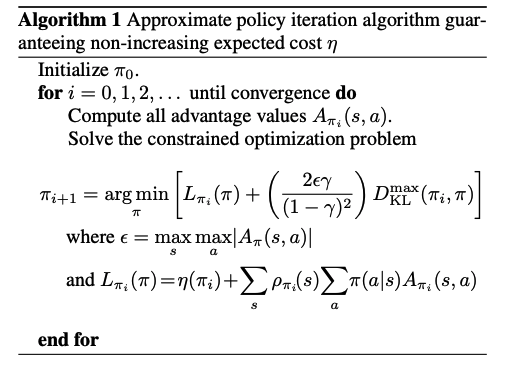## 0x09 Actor-Critic及变种

### Actor-Critic 方法

Actor网络用于选择动作以达到最大的回报，Critic网络用于对动作进行打分，以减少回报估计值和回报真实之间的差距为目标。

$\Delta \theta = \alpha \nabla_{\theta} log\pi_{\theta}(a|s)Q_{w}(s,a)$

• 使用梯度上升更新Critic网络：

$\delta = r + \gamma Q_w(s',a')-Q_w(s,a)\\ w = w + \beta\delta\nabla_wQ_w(s,a)$

• 使用梯度下降更新Actor网络：

$\theta = \theta+\alpha \nabla_{\theta} log\pi_{\theta}(a|s)Q_{w}(s,a)$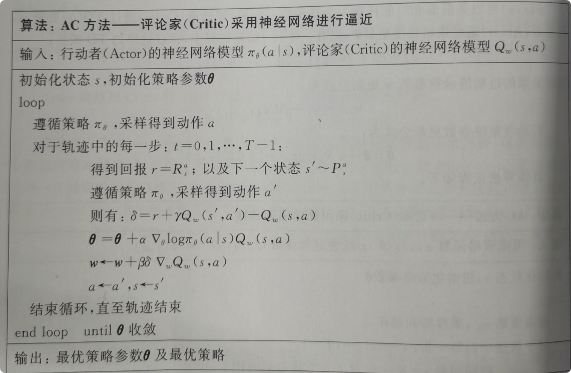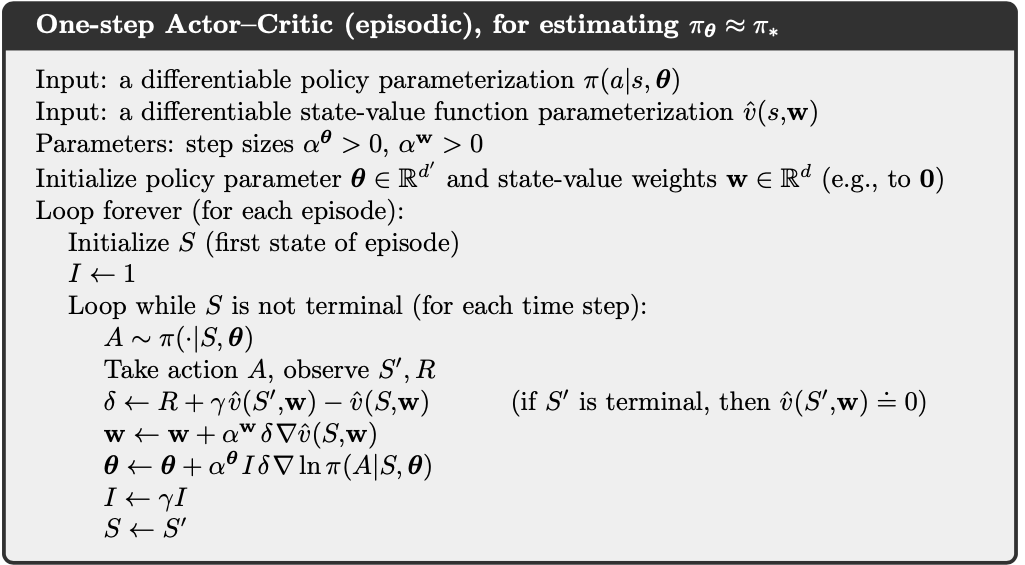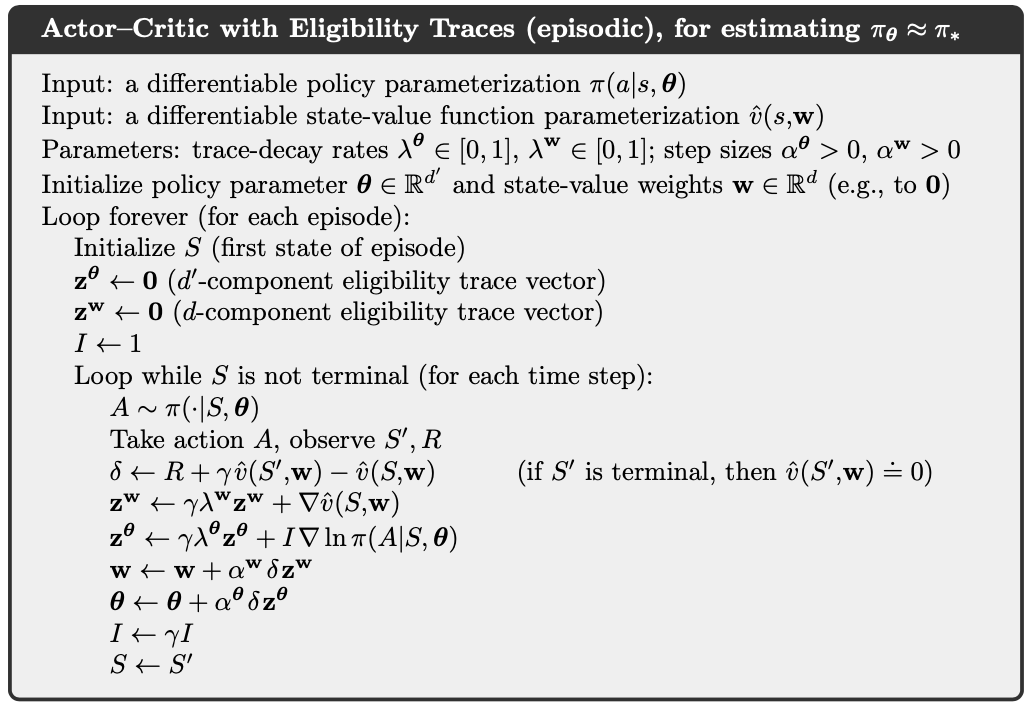$\nabla_{\theta}J(\theta)=E_{\pi_{\theta}}[\nabla_{\theta}log\pi_{\theta}(a|s)(Q_{\pi_{\theta}}(s,a)-V_{\pi_{\theta}}(s))]=E_{\pi_{\theta}}[\nabla_{\theta}log\pi_{\theta}(a|s)A_{\pi_{\theta}}(s,a)]$

$\delta_{\pi_{\theta}} = r+\gamma V_{\pi_{\theta}}(s')-V_{\pi_{\theta}}(s)\\ E_{\pi_{\theta}}[\delta_{\pi_{\theta}}|s,a] = E_{\pi_{\theta}}[r+\gamma V_{\pi_{\theta}}(s')|s,a]-V_{\pi_{\theta}}(s)=Q_{\pi_{\theta}}(s,a)-V_{\pi_{\theta}}(s)=A_{\pi_{\theta}}(s,a)$

$\nabla_{\theta}J(\theta)=E_{\pi_{\theta}}[\nabla_{\theta}log\pi_{\theta}(a|s)\delta_{\pi_{\theta}}]$